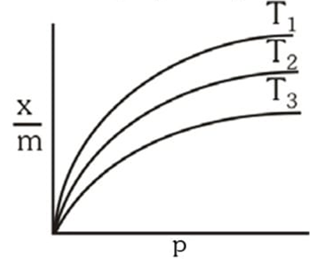Show below are adsorption isotherms for a gas ‘X’ at temperatures T1,  T2  and  T3 :p and x/m represent pressure and extent of adsorption, respectively. The correct order of temperatures for the given adsorption is :

# Show below are adsorption isotherms for a gas ‘X’ at temperatures ${\mathrm{T}}_{1},\text{\hspace{0.17em}\hspace{0.17em}}{\mathrm{T}}_{2}\text{\hspace{0.17em}\hspace{0.17em}}\mathrm{and}\text{\hspace{0.17em}\hspace{0.17em}}{\mathrm{T}}_{3}$ :p and x/m represent pressure and extent of adsorption, respectively. The correct order of temperatures for the given adsorption is :

1. A

${T}_{1}>\text{\hspace{0.17em}\hspace{0.17em}}{T}_{2}\text{\hspace{0.17em}}>\text{\hspace{0.17em}\hspace{0.17em}}{T}_{3}$

2. B

${T}_{3}>\text{\hspace{0.17em}\hspace{0.17em}}{T}_{2}\text{\hspace{0.17em}}>\text{\hspace{0.17em}\hspace{0.17em}}{T}_{1}$

3. C

${T}_{1}=\text{\hspace{0.17em}\hspace{0.17em}}{T}_{2}\text{\hspace{0.17em}}=\text{\hspace{0.17em}\hspace{0.17em}}{T}_{3}$

4. D

${T}_{1}=\text{\hspace{0.17em}\hspace{0.17em}}{T}_{2}\text{\hspace{0.17em}}>\text{\hspace{0.17em}\hspace{0.17em}}{T}_{3}$

Fill Out the Form for Expert Academic Guidance!l

+91

Live ClassesBooksTest SeriesSelf Learning

Verify OTP Code (required)

### Solution:

Adsorption is exothermic process. According to lechatlier's principal exothermic process are favourable for low temperature.

With increase in temperature x/m decreases. so, correct order of
temperature is  ${\mathrm{T}}_{3}>\text{\hspace{0.17em}\hspace{0.17em}}{\mathrm{T}}_{2}\text{\hspace{0.17em}}>\text{\hspace{0.17em}\hspace{0.17em}}{\mathrm{T}}_{1}$

## Related content

 Differences & Comparisons Articles in Chemistry Magnesium oxide Formula Barium chloride formula Benzoic Acid formula Butane Formula Magnesium Chloride Formula Magnesium hydroxide Formula Nitrite Formula Phosphoric Acid Formula Salicylic Acid Formula+91

Live ClassesBooksTest SeriesSelf Learning

Verify OTP Code (required)